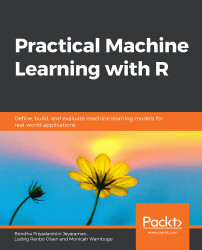•#### Practical Machine Learning with R#### Overview of this book

With huge amounts of data being generated every moment, businesses need applications that apply complex mathematical calculations to data repeatedly and at speed. With machine learning techniques and R, you can easily develop these kinds of applications in an efficient way. Practical Machine Learning with R begins by helping you grasp the basics of machine learning methods, while also highlighting how and why they work. You will understand how to get these algorithms to work in practice, rather than focusing on mathematical derivations. As you progress from one chapter to another, you will gain hands-on experience of building a machine learning solution in R. Next, using R packages such as rpart, random forest, and multiple imputation by chained equations (MICE), you will learn to implement algorithms including neural net classifier, decision trees, and linear and non-linear regression. As you progress through the book, you’ll delve into various machine learning techniques for both supervised and unsupervised learning approaches. In addition to this, you’ll gain insights into partitioning the datasets and mechanisms to evaluate the results from each model and be able to compare them. By the end of this book, you will have gained expertise in solving your business problems, starting by forming a good problem statement, selecting the most appropriate model to solve your problem, and then ensuring that you do not overtrain it.Free Chapter
An Introduction to Machine LearningData Cleaning and Pre-processingFeature EngineeringIntroduction to neuralnet and Evaluation MethodsLinear and Logistic Regression ModelsUnsupervised LearningAppendix## Introduction

Data cleaning and preparation takes about 70% of the effort in the entire process of a machine learning project. This step is essential because the quality of the data determines the accuracy of the prediction model. A clean dataset should contain good samples of the scenarios that we want to predict, and this will give us good prediction results. Also, the data should be balanced, which means that every category we want to predict should have similar number of samples. For example, if we want to predict whether it will rain or not on any particular day, and if the sample data size is 100, the data could contain 40 samples for It will rain and 60 samples for It will not rain today, or vice versa. However, if the ratio is 20:80 or 30:70, it is an unbalanced dataset, and this will not yield good results for the minority class.

In the following section, we will look at the essential operations performed on data frames in R. These operations will help us to manipulate and analyze...